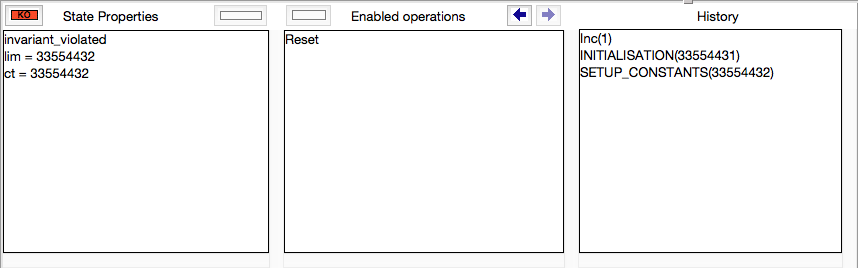• Special Pages
• User

Help

# Bounded Model Checking

(diff) ← Older revision | Latest revision (diff) | Newer revision → (diff)

As of version 1.5, ProB provides support for constraint-based bounded model checking (BMC). As opposed to constraint-based checking, BMC finds counterexamples which are reachable from the INITIALISATION. As opposed to ordinary model checking, it does not compute all possible solutions for parameters and constants, but constructs these on demand. This can be useful when the out-degree of the state-space is very high, i.e., when there are many possible solutions for the constants, the initialisation and/or the individual operations and their parameters.

Take the following example:

```MACHINE VerySimpleCounterWrong
CONSTANTS lim
PROPERTIES lim = 2**25
VARIABLES ct
INVARIANT
ct:INTEGER & ct < lim
INITIALISATION ct::0..(lim-1)
OPERATIONS
Inc(i) = PRE i:1..1000 & ct + i <= lim THEN ct := ct+i END;
Reset = PRE ct = lim THEN ct := 0 END
END
```

The ProB model checker will here run for a very long time before uncovering the error that occurs when ct is set to lim. If you run the TLC backend you will get the error message "Too many possible next states for the last state in the trace."

However, for the bounded model checker this is less of a problem; it will use the constraint solver to try and find suitable values of the constants, initial variable values and parameters to generate an invariant violation. The bounded model checker actually uses the same algorithm than the constraint-based test-case generator, but uses the negation of the invariant as target. You can use the command-line version of ProB, with the -bmc DEPTH command as follows:

```\$ probcli VerySimpleCounterWrong -bmc 10
constraint based test case generation, maximum search depth: 10
constraint based test case generation, target state predicate: #not_invariant
constraint based test case generation, output file:
constraint based test case generation, performing bounded model checking
bounded_model_checking

CBC test search: starting length 1
*1.!#
CBC test search: covered event Inc
Found counter example
executing_stored_test_case(1,[Inc],[(2,Inc(int(1)),1,2)])
finding_trace_from_to(root)

BMC counterexample found, Length=1

CBC Algorithm finished (1)
Timeouts: total: 0
Checked paths (Depth:Paths): 1:1, total: 1
Found paths: 1:1, total: 1
Found test cases: 1:1, total: 1
Runtime (wall): 120 ms
! An error occurred !
! source(invariant_violation)
! Invariant violation found by bmc
! *** error occurred ***
! invariant_violation
```

You can also use the bounded model checker from ProB Tcl/Tk. The command is situated inside the Experimental sub-menu of the Analyse menu (to see the command, you may have to change the preference entry to preference(user_is_an_expert_with_accessto_source_distribution,true). in the ProB_Preferences.pl file) Running the command on the above example will generate the following counter-example:

``````